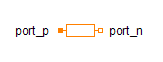Constant Vector - MapleSim Help

Constant Vector

Generate constant n-vector signal of type RealDescription The Constant Vector component has a real output vector equal to the real vector parameter $k$.Equations $y=k$Connections

 Name Description Modelica ID $y$ Real output signals yParametersGeneral Parameters

 Name Default Units Description Modelica ID $k$ $\left[1\right]$ Vector output value kModelica Standard Library The component described in this topic is from the Modelica Standard Library. To view the original documentation, which includes author and copyright information, click here.